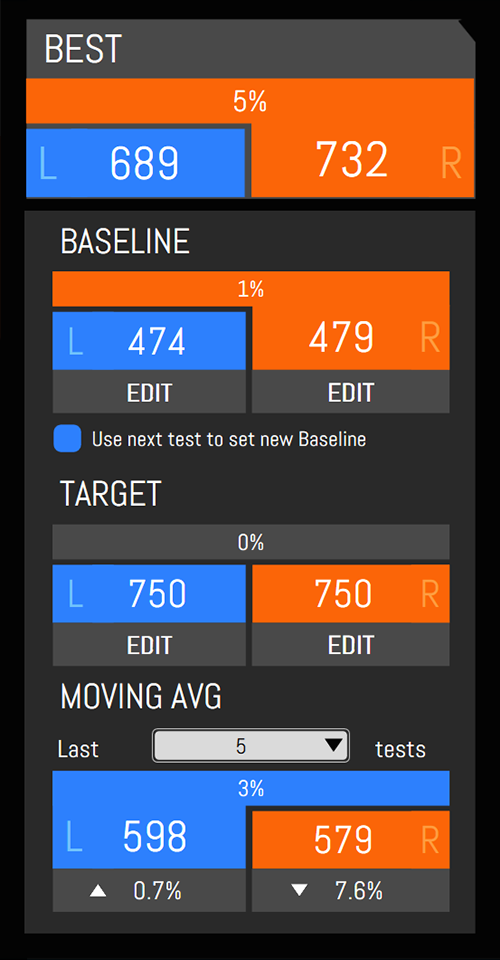# Understanding NordBord Results in the ScoreBord App

When you run a NordBord test in ScoreBord, a number of metrics are calculated automatically.  Here's a rundown of what the on-screen metrics mean.

Related:   Understanding ForceFrame Results in the ScoreBord App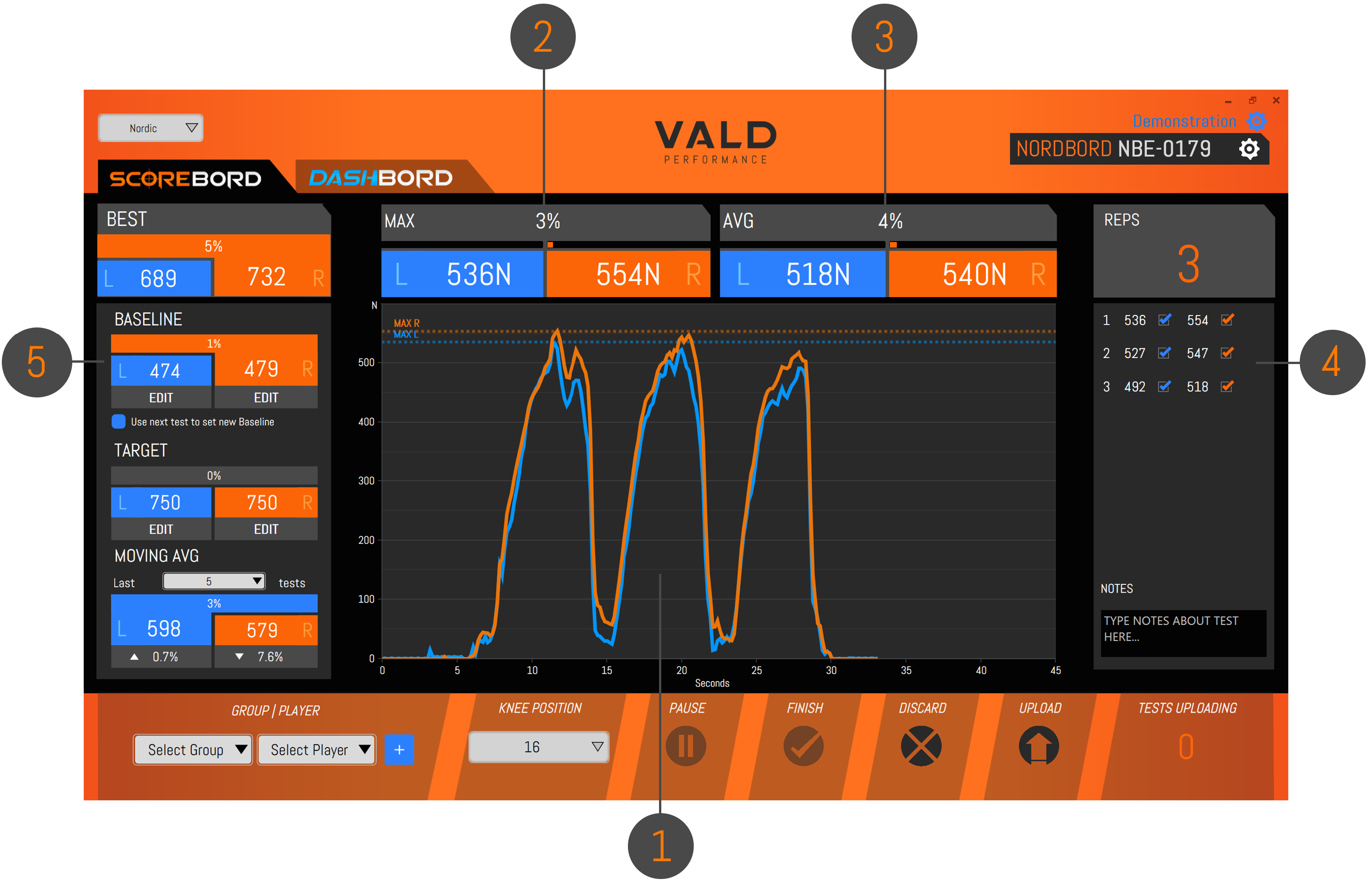1 The main graphing window shows a force-time trace during a test (i.e. Y-axis = force in newtons, and Y-axis = time in seconds): Y-axis = force in newtons (N) X-axis = time in seconds (s) Blue line = left leg force Orange line = right leg force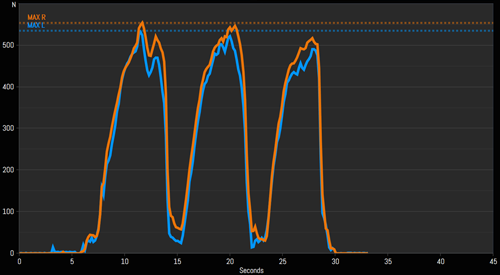2 The top-left results panel shows maximum strength results: Maximum left leg force is shown in blue Maximum right leg force is shown in orange Imbalance (i.e. percentage difference between left and right maximums) is shown in grey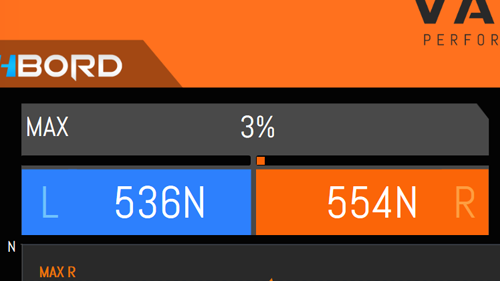3 The top-right results panel shows average strength results: Average left leg force is shown in blue Average right leg force is shown in orange Imbalance (i.e. percentage difference between left and right averages) is shown in grey Note: "Average" is the average of the peaks of all reps performed during the test.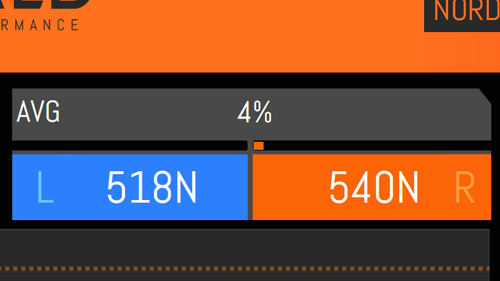4 The right-hand results bar shows rep-by-rep results for left and right leg. Unwanted reps (e.g. accidental reps or reps performed with undesireable form) can be de-selected using the checkboxes next to each rep.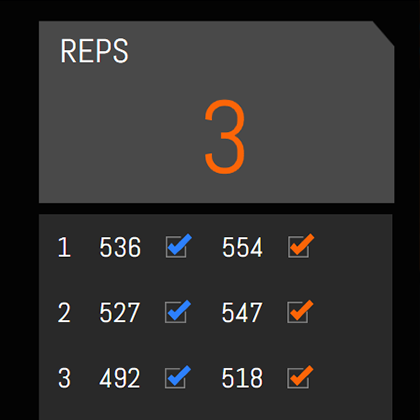5 The left-hand results bar shows reference points for the person being tested, in the test type being conducted:  Best is their highest ever left and right leg results for that test Baseline is an editable reference point (often recorded at the start of a preseason or season) Target is an editable goal for the person to aim for Moving Avg is the average result from the last 1-10 tests